# Here’s the gist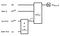The general structure of QGANs. The real source R or the parametrized generator G(theta_G) is applied on an initial state |0⟩, and each outputs a mixed or pure quantum state described by a density matrix. The discriminator is denoted as D(theta_D). We will discuss details on each register later.

## A quick note before we jump into the fun stuff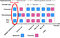Timeline of the development of quantum generative adversarial network models (source). The implementation we’ll be covering (circled in red) was first proposed by Dallaire-Demers et al. (source), and several concepts in this article are drawn from that original paper.

# Let’s dive a bit deeper.

## The cost function — for the mathematically motivated

`Z = |0⟩⟨0| - |1⟩⟨1|  # when we label |real⟩ as |0⟩ and |fake⟩ as |1⟩ 〈real|Z|real〉= 1  # Expvals of statevectors with respect to Z are〈fake|Z|fake〉= −1   proportional to the correct classification`The real source R or G(θ_G) is applied on the initial state |0,z⟩ respectively defined on the Out R|G and Bath R|G registers. The discriminator uses the outputted state from the source and an initial state |0,0⟩ defined on the Out D, and Bath D registers to output its’ answer |real⟩ or |fake⟩ in the Out D register. Bath D and Bath R|G are workspaces for the discriminator and generator, respectively.Eq 4 — recall that the expectation value of a density matrix with respect to an observable Z is the trace of that Z operator applied to the density matrix. Read here to learn more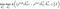The final quantum optimization problem for QGANs

## The algorithmic flow summed up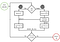The algorithmic flow of a QGAN, source: Dallaire-Demers et al. (2018)

# Some thoughts to keep in mind

## An ansatz suggestion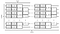A practical universal circuit ansatz for either G or D. Each layer is composed of parameterized single-qubit X rotations followed by parameterized Z rotations. A layer of staggered sets of parameterized nearest-neighbour ZZ rotations follows the single-qubit rotations.

# Wrapping it up

## Resources

17 y/o Quantum Machine Learning Fanatic | QHack top 15 | Financial markets |

## More from Pavan Jayasinha

17 y/o Quantum Machine Learning Fanatic | QHack top 15 | Financial markets |